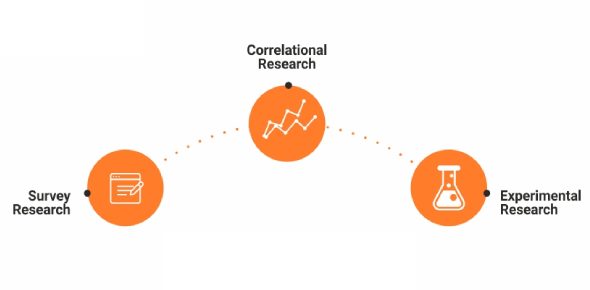# Quantitative Research Designs Quiz Questions

20 Questions | Attempts: 3391
ShareSettings.

• 1.
Which of the following is TRUE about features of quasi-experimental research design?
• A.

Manipulation. control group, randomization

• B.

Manipulation, but no control group or randomization

• C.

No manipulation of independent variable

• D.

Use of correlational approach

• 2.
Carry-over effects are related to:
• A.

Factorial design

• B.

Pretest-posttest design

• C.

Solomon four-group design

• D.

Repeated measures design

• 3.
The extent to which it is possible to make an inference that the independent variable is truly influencing the dependent variable and relationship is not spurious, refers to:
• A.

External validity

• B.

Internal Validity

• C.

Internal consistency

• D.

Reliability

• 4.
Which of the following is considered as a threat to internal validity of a research?
• A.

The Hawthorne effect

• B.

Novelty effect

• C.

Experimenter effects

• D.

Maturation

• E.

Measurement effects

• 5.
Which of the following is NOT related to dealing with extraneous variables in a quantitative research?
• A.

Randomization

• B.

Repeated measures

• C.

Homogeneity

• D.

Blocking

• E.

Bracketing

• 6.
Which of the following is a method of integrating the findings of prior research studies using statistical procedures?
• A.

Meta-analysis

• B.

Secondary analysis

• C.

Content analysis

• D.

Grounded theory

• 7.
The term used to indicate the placebo effect in which the knowledge of being included in a study causes people to change their behaviors, thereby obscuring the variable of interest:
• A.

Internal validity

• B.

Maturation

• C.

Manipulation

• D.

Hawthorne effect

• 8.
Investigations in which samples from a general population are studied over time with respect to some phenomenon is termed as
• A.

Trend studies

• B.

Action research

• C.

Outcome research

• D.

Questionnaires

• 9.
Which of the following data collection method has better subjectivity?
• A.

Self-reports

• B.

Observation

• C.

Biophysiologic measures

• D.

Questionnaires

• 10.
Basic principles that are accepted as being true on the basis of logic or reason, without proof or verification is termed as:
• A.

Assumptions

• B.

Hypothesis

• C.

Constructs

• D.

Propositions

• 11.
A fundamental ethical principle that seeks to prevent harm and exploitation of, to maximize benefits for, study participants is:
• A.

Justice

• B.

Beneficence

• C.

Nonmalificence

• D.

Coercion

• 12.
A method of data collection in which several rounds of questionnaires are mailed to a panel of experts, focusing on their opinions or judgment concerning a specific topic of interest is:
• A.

Focus group interview

• B.

Meta-analysis

• C.

Delphi technique

• D.

Secondary analysis

• 13.
All of the following diagrams can be draw from quantitative data, except:
• A.

Frequency curve

• B.

Scatter diagram

• C.

Flow diagram

• D.

Histogram

• 14.
Best graph for demonstration of relationship between ages and weight is:
• A.

Bar Diagram

• B.

Histogram

• C.

Scatter

• D.

Pie diagram

• 15.
The phenomenon of those who obtain extreme scores at pretest tending to obtain less extreme scores at posttest, at both the high and low ends of the measure is:
• A.

Internal validity

• B.

Statistical regression

• C.

Statistical power

• D.

Statistical validity

• 16.
The probability that a given effect will be detected using a sample of a specified size, with the probability level of the statistical test being set at a predetermined level is:
• A.

Power of a statistical test

• B.

Internal validity

• C.

External validity

• D.

Reliability of a study

• 17.
The probability of making a Type II error in any statistical testis termed as:
• A.

Alpha

• B.

Beta

• C.

Gamma

• D.

Theta

• E.

Delta

• 18.
A pharmacology trial concluded that a new antipsychotic reduced negative symptoms when in reality it did not; is an example of:
• A.

Type I error

• B.

Type II error

• C.

Type III error

• D.

Type IV error

• 19.
The sum of the values of a variable for a set of observations, divided by the number of the observations in the set refers to:
• A.

Variance

• B.

Standard deviation

• C.

Median

• D.

Mean

• E.

Mode

• 20.
Which of the following is NOT a multivariate statistical approach?
• A.

Analysis of Covariance (ANCOVA)

• B.

Multiple Regression

• C.

Factor Analysis

• D.

Structural Equation Modeling

• E.

Analysis of Variance

## Related TopicsBack to top
×

Wait!
Here's an interesting quiz for you.